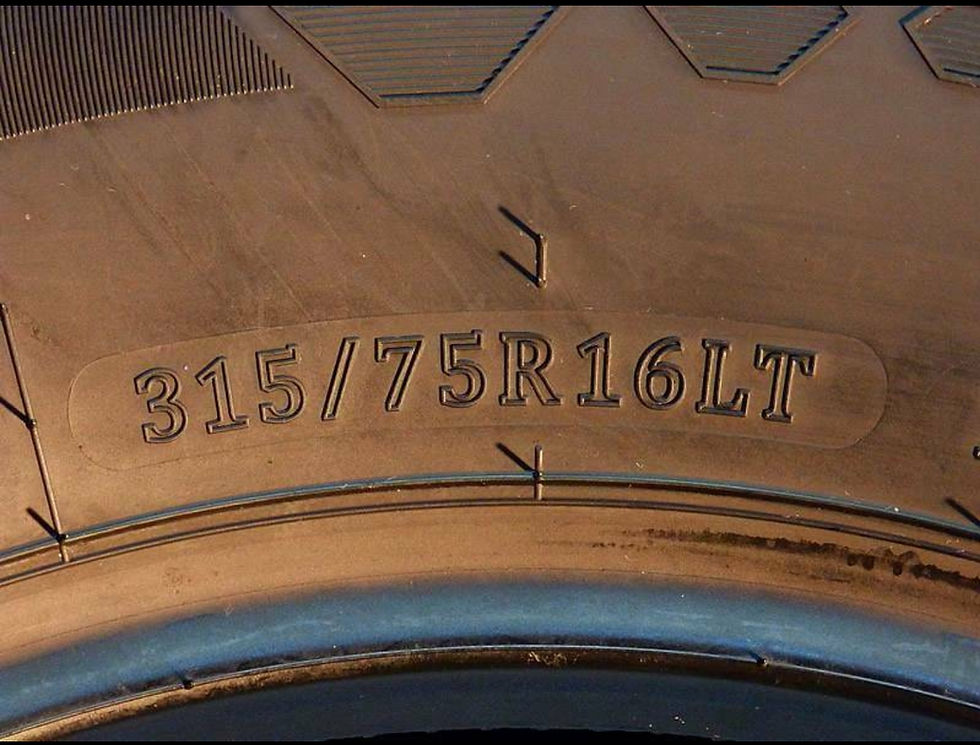top of page
Search

# The easy way to work out your tyre size.

Updated: Mar 20, 2022You probably have heard 4-wheel drivers talk about have "35s" or "33s", referring to wheel and tyre size. Maybe that's the language you speak. But do you know how to work this out? It is really simple primary school mathematics. This little formula can help you work it out for yourself in a few simple stepsOn the side of a tyre there will be some numbers indicating the tyre size. For example lets work with 315/75x16.

Here is how to use them:

Step 1

The number '315' refers to the width of the tyre in mm (see A).Step 2

The number '75' is a percentage, and refers to the profile of the tyre. It means that the tyre wall is 75% of the tyre's width. So 315 x 0.75 = 236.25mm (see B).

Step 3

Then add the wheel rim diameter (see C). That is the last number - in the above example it is '16'. The tricky part is this figure is in inches, and you need to multiply it by 25.4 to convert it to mm. So 16 x 25.4 = 405.4mm.

Step 4

So now we add up the three components:

(236.25 x 2) + 405.4 = 877.9mm

To get this back into inches we divide 877.9mm by 25.4 = 34.56 inches or 34.5s. Simple!

Or put into one simple equation it is:

2(315x0.75) + (16x25.4) ÷ 25.4 = Wheel size in inches

or 2(width mm x profile) + (wheel diameter inches x 25.4) ÷ 25.4